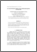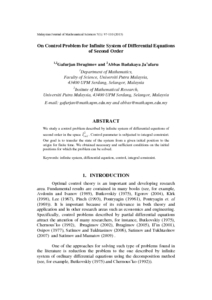# On control problem for infinite system of differential equations of second order

## Citation

Ibragimov, Gafurjan and Ja’afaru, Abbas Badakaya (2013) On control problem for infinite system of differential equations of second order. Malaysian Journal of Mathematical Sciences, 7 (1). pp. 97-110. ISSN 1823-8343

## Abstract

We study a control problem described by infinite system of differential equations of second order in the space 2 r 1l+. Control parameter is subjected to integral constraint. Our goal is to transfer the state of the system from a given initial position to the origin for finite time. We obtained necessary and sufficient conditions on the initial positions for which the problem can be solved.Preview
PDF
30169.pdfView Item